Here is a jumpstart for this exercise. It took a bit of doing to get the table laid out nicely, so I have supplied the source code!

 1. Consider the following sequence of patterns:Which of the following patterns comes next? A, B, C, D, E, or F: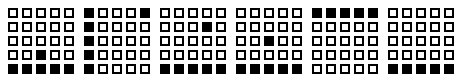A B C D E F 2. Consider the following sequence of patterns: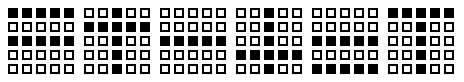Which of the following patterns comes next? A, B, C, D, E, or F: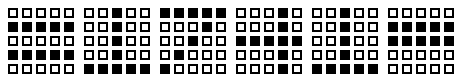A B C D E F 3. Consider the following sequence of patterns: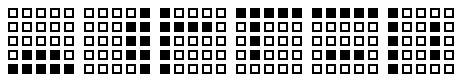Which of the following patterns comes next? A, B, C, D, E, or F:A B C D E F 4. Consider the following sequence of patterns:Which of the following patterns comes next? A, B, C, D, E, or F: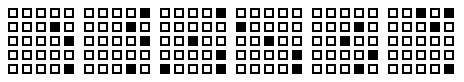A B C D E F 5. Consider the following sequence of patterns: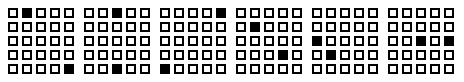Which of the following patterns comes next? A, B, C, D, E, or F: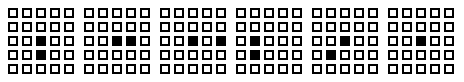A B C D E F 6. Consider the following sequence of patterns: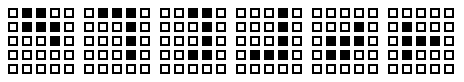Which of the following patterns comes next? A, B, C, D, E, or F:A B C D E F 7. Consider the following sequence of patterns: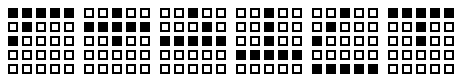Which of the following patterns comes next? A, B, C, D, E, or F:A B C D E F 8. Consider the following sequence of patterns:Which of the following patterns comes next? A, B, C, D, E, or F: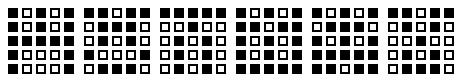A B C D E F

1 Answer C : 2 Answer F : 3 Answer B : 4 Answer A : 5 Answer C : 6 Answer B : 7 Answer A : 8 Answer C